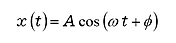FFT analyzer FAQReading line spectrum values for a given frequency at peak value (or as P-P values)Because a single-line value in a power spectrum (i.e., frequency bands) is expressed as a mean square, its linear spectrum is a root mean square value, but because the analytical range (frequency resolution) in FFT analysis is small, its output can resemble a sine wave (or cosine wave). Consequently, its frequency amplitude (peak value, half amplitude) can be approximated as the square root of the root mean square. This approximate peak value is also sometimes called the equivalent peak value. Similarly, the P-P value (peak-to-peak value, double amplitude) can be determined as the square root of the root mean square times two (2√2).

For example,when the input signal is A, the root mean square is A/√2, the amplitude (peak value) is A, and the P-P value is 2A.

Revised:2009/11/16